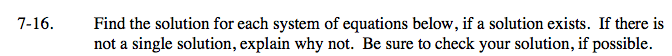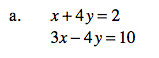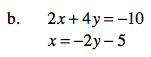Home > CAAC > Chapter 7 > Lesson 7.1.2 > Problem7-16

7-16.
1. Find the solution for each system of equations below, if a solution exists. If there is not a single solution, explain why not. Be sure to check your solution, if possible. Homework Help ✎

1. x + 4y = 2
3x − 4y = 10

2. 2x + 4y = −10
x = −2y − 5For help solving systems of equations, see problem 6-71.(3, −0.25)